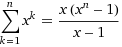SEARCH HOMEMath Central Quandaries & QueriesQuestion from Tim, a student: pretend I have a password that is - 9k7d4112p5o assuming that you continuously guessed wrong until that exact combination was the only possible answer left; how many times would you have to guess? capital letters are not relevant and you do not know how many digits there areHi Tim. I have to make some assumptions to give you a numerical answer.

First assumption: password characters can be any English alphabetic character or any numeric character (no symbols, spaces, etc). So that means there are 26+10 choices for each character.

Second assumption: Your password is as long as it is allowed to be. That means that since 9k7d4112p5o is 11 characters long, then passwords must be at least 1 character and at most 11 characters long.

There are 36 possible one-character passwords.
There are 362 possible two-character passwords.
There are 363 possible three-character passwords.
and so on.

So the next question is this:
What is 36 + 362 + 363 + ... + 3611 ?

I won't show you the derivation (you could just use your calculator too), but the sum like this can be simplified to:So the number of guesses is 36(3611 - 1)/35 which my calculator says is 135 382 323 952 046 196 or about 135 quadrillion. That would take some time.

Cheers,
Steve La RocqueMath Central is supported by the University of Regina and The Pacific Institute for the Mathematical Sciences.